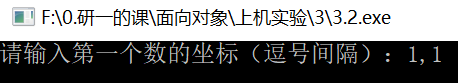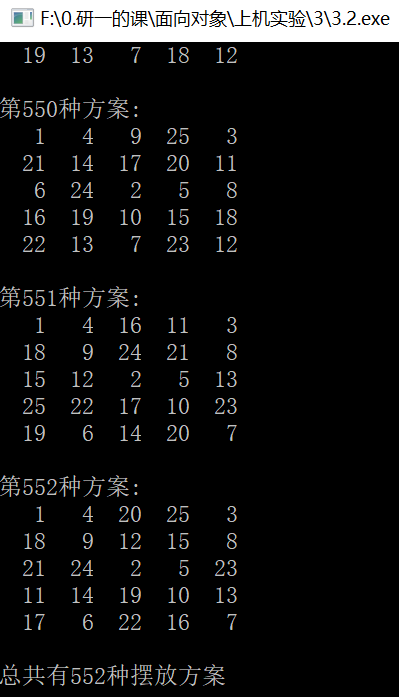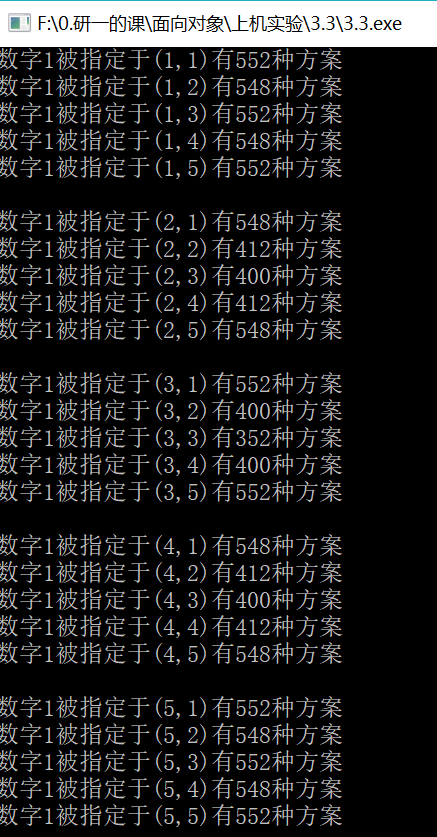# 【算法】矩阵填数，深度优先搜索（DFS），Pascal改C语言

### 题目

1）（E, W）=（x±3，y）

2）（E, W）=（x，y±3）

3）（E, W）=（x±2，y±2）

### 参考答案

 1 Program lx9_1_3;
2 uses crt;
3 const n=5;
4       d:array[1..8，1..2] of shortint=((3，0)，(-3，0)，(0，3)，(0，-3)，
5                                       (2，2)，(2，-2)，(-2，2)，(-2，-2));
6 var x0，y0:byte;
7     a:array[1..n，1..n] of byte;
8     total:longint;
9
10 procedure print;
11   var i，j:integer;
12   begin
13     inc(total);
14     gotoxy(1，3);
15     writeln('['，total，']');
16     for i:=1 to n do
17       begin
18         for j:=1 to n do
19           write(a[i，j]:3);
20         writeln;
21       end;
22   end;
23
24 procedure try(x，y，k:byte);
25   var i，x1，y1:integer;
26   begin
27     for i:=1 to 8 do
28       begin
29         x1:=x+d[i，1];y1:=y+d[i，2];
30         if (x1>0) and (y1>0) and (x1<=n)
31            and (y1<=n) and (a[x1，y1]=0) then
32              begin
33                a[x1，y1]:=k;
34                if k=n*n then print
35                   else try(x1，y1，k+1);
36                a[x1，y1]:=0;
37              end;
38       end;
39   end;
40
41 begin
42   clrscr;
44   fillchar(a，sizeof(a)，0);
45   total:=0;a[x0，y0]:=1;
46   try(x0，y0，2);
47   writeln('Total='，total);
48   writeln('Press any key to exit..。');
49   repeat until keypressed;
50 end.

### 自改C语言代码

 1 #include <stdio.h>
2 #define N 5//格子行列数
3
4 int next={{3,0},{-3,0},{0,3},{0,-3},{2,2},{2,-2},{-2,2},{-2,-2}};//下一步变换的位移
5 int x0,y0;//输入的初始坐标
6 int matrix[N][N];//存储nxn矩阵中的数字
7 int total;//方案数量
8
9 //打印一个矩阵的函数
10 void printMatrix(){
11     int i,j;
12     total+=1;//出来一次结果，就打印一次，方案数+1
13     printf("第%d种方案:\n",total);
14     for(i=0;i<N;i++){
15         for(j=0;j<N;j++){
16             printf("%4d",matrix[i][j]);//%4d表示输出宽度为4，且右对齐
17         }
18         printf("\n");
19     }
20     printf("\n");
21 }
22 ////每个初始点都输出方案的个数的话就不把每个方案打印出来了，太占地方
23 //void printMatrix(){
24 //    int i,j;
25 //    total+=1;//出来一次结果，就打印一次，方案+1
26 //}
27
28 //主要函数,(x,y)是矩阵内坐标,k是第几个数字
29 void try(x,y,k){
30     int i,x1,y1;//x1,y1是本次要找的坐标
31     //将8个位移都试一遍
32     for(i=0;i<8;i++){
33         x1=x+next[i];
34         y1=y+next[i];
35         //如果该位置不超过边界且没有数字，则方案可行，把数字装进这个位置
36         if((x1>-1)&&(y1>-1)&&(x1<N)&&(y1<N)&&(matrix[x1][y1]==0)){
37             matrix[x1][y1]=k;
38             //如果k=25即已经搜索完，可以打印了
39             if(k==N*N)
40                 printMatrix();
41             //如果还没到25，就继续搜索下一个位置
42             else
43                 try(x1,y1,k+1);
44             //本次打印完/本次搜索尝试到死路，回溯到上一节点去往另一分支前，将这一节点清零
45             matrix[x1][y1]=0;
46         }
47     }
48 }
49
50
51 int main()
52 {
53     //输入坐标
54     printf("请输入第一个数的坐标（逗号间隔）：");
55     scanf("%d,%d",&x0,&y0);
56
57     //矩阵整体清0（第一条在dev-c++可以跑,vc++不行，还是用老办法）
58     //memset(matrix, 0, sizeof(matrix));
59     int i,j;
60     for(i=0;i<N;i++){
61         for(j=0;j<N;j++){
62             matrix[i][j]=0;
63         }
64     }
65
66     //数据初始化，运行
67     total=0;//方案数为零
68     x0-=1;y0-=1;//用户输入坐标从1开始，c语言数组从0开始
69     matrix[x0][y0]=1;//第一个位置赋值1
70     try(x0,y0,2); //从数字2开始放置
71
72     printf("总共有%d种摆放方案\n\n",total);
73
74
75 //    //每个初始点都输出方案的个数
76 //    int m,n;
77 //    for(m=0;m<5;m++){
78 //        for(n=0;n<5;n++){
79 //                x0=m;y0=n;
80 //                //矩阵整体清0
81 //                int i,j;
82 //                for(i=0;i<N;i++){
83 //                    for(j=0;j<N;j++){
84 //                        matrix[i][j]=0;
85 //                    }
86 //                }
87 //                total=0;//每次节点前方案数清零
88 //                matrix[x0][y0]=1;//第一个位置赋值1
89 //                try(x0,y0,2); //从数字2开始放置
90 //                printf("数字1被指定于(%d,%d)有%d种方案\n",m+1,n+1,total);
91 //        }
92 //        printf("\n");//每5行之后打个空行好看点
93 //    }
94 }

### 结果

• 输入一个起始点坐标后输出的方案• 输出所有起始点的方案个数：posted @ 2019-10-24 22:44  threells1939  阅读(...)  评论(...编辑  收藏